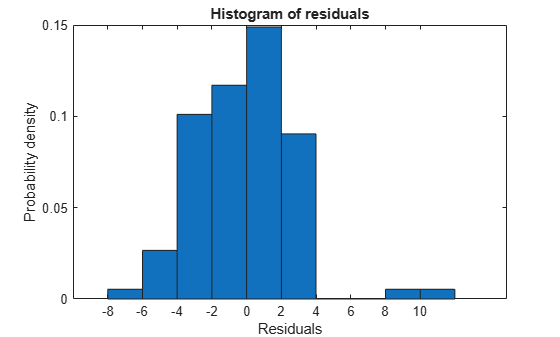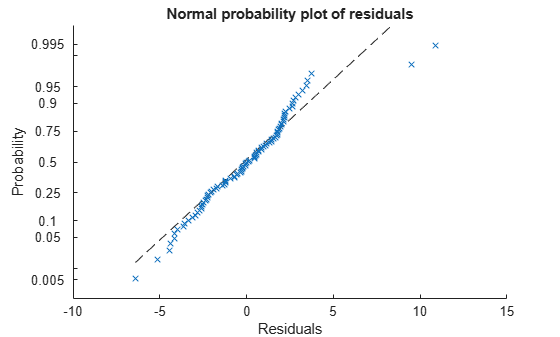plotResiduals

Plot residuals of linear regression model

Description

example

plotResiduals(mdl) creates a histogram plot of the linear regression model (mdl) residuals.

example

plotResiduals(mdl,plottype) specifies the residual plot type plottype.

plotResiduals(mdl,plottype,Name,Value) specifies additional options using one or more name-value pair arguments. For example, you can specify the residual type and the graphical properties of residual data points.

plotResiduals(ax,___) creates the plot in the axes specified by ax instead of the current axes, using any of the input argument combinations in the previous syntaxes.

h = plotResiduals(___) returns graphics objects for the lines or patch in the plot. Use h to modify the properties of a specific line or patch after you create the plot. For a list of properties, see Line Properties and Patch Properties.

Examples

collapse all

Plot a histogram of the residuals of a fitted linear regression model.

Load the carsmall data set and fit a linear regression model of the mileage as a function of model year, weight, and weight squared.

tbl = table(MPG,Weight);
tbl.Year = categorical(Model_Year);
mdl = fitlm(tbl,'MPG ~ Year + Weight^2');

Create a histogram of the raw residuals using probability density function scaling.

plotResiduals(mdl)The area of each bar is the relative number of observations. The sum of the bar areas is equal to 1.

Create a normal probability plot of the residuals of a fitted linear regression model.

Load the carsmall data set and fit a linear regression model of the mileage as a function of model year, weight, and weight squared.

X = [Weight,Model_Year];
mdl = fitlm(X,MPG,...
'y ~ x2 + x1^2','Categorical',2);

Create a normal probability plot of the residuals of the fitted model.

plotResiduals(mdl,'probability')Input Arguments

collapse all

Linear regression model, specified as a LinearModel object created using fitlm or stepwiselm.

Plot type, specified as one of the values in this table.

ValueDescription
'caseorder'Residuals vs. case order (row number)
'fitted'Residuals vs. fitted values
'histogram'Histogram of residuals using probability density function scaling. The area of each bar is the relative number of observations. The sum of the bar areas is equal to 1.
'lagged'Residuals vs. lagged residuals (r(t) vs. r(t – 1))
'probability'Normal probability plot of residuals. For details, see probplot.
'symmetry'Symmetry plot of residuals around their median (residuals in upper tail – median vs. median – residuals in lower tail). This plot includes a dotted reference line of y = x to examine the symmetry of residuals.

Target axes, specified as an Axes object.

If you do not specify the axes and the current axes are Cartesian, then plotResiduals uses the current axes (gca). For more information on creating an Axes object, see axes and gca.

Name-Value Arguments

Specify optional comma-separated pairs of Name,Value arguments. Name is the argument name and Value is the corresponding value. Name must appear inside quotes. You can specify several name and value pair arguments in any order as Name1,Value1,...,NameN,ValueN.

Example: 'Color','blue','Marker','o'

Note

The graphical properties listed here are only a subset. For a complete list, see Line Properties for lines and Patch Properties for histogram. The specified properties apply to the appearance of residual data points or the appearance of the histogram.

Type of residual used in the plot, specified as the comma-separated pair consisting of 'ResidualType' and one of these values:

ValueDescription
'raw'Observed minus fitted values
'pearson'Raw residuals divided by the root mean squared error (RMSE)
'standardized'Raw residuals divided by their estimated standard deviation
'studentized'Raw residuals divided by an independent (delete-1) estimate of their standard deviation

The Residuals property of mdl contains the residual values used by plotResiduals to create plots.

For details, see Residuals.

Example: 'ResidualType','Pearson'

Line color, specified as the comma-separated pair consisting of 'Color' and an RGB triplet, hexadecimal color code, color name, or short name for one of the color options listed in the following table.

The 'Color' name-value pair argument also determines marker outline color and marker fill color if 'MarkerEdgeColor' is 'auto' (default) and 'MarkerFaceColor' is 'auto'.

For a custom color, specify an RGB triplet or a hexadecimal color code.

• An RGB triplet is a three-element row vector whose elements specify the intensities of the red, green, and blue components of the color. The intensities must be in the range [0,1]; for example, [0.4 0.6 0.7].

• A hexadecimal color code is a character vector or a string scalar that starts with a hash symbol (#) followed by three or six hexadecimal digits, which can range from 0 to F. The values are not case sensitive. Thus, the color codes '#FF8800', '#ff8800', '#F80', and '#f80' are equivalent.

Alternatively, you can specify some common colors by name. This table lists the named color options, the equivalent RGB triplets, and hexadecimal color codes.

Color NameShort NameRGB TripletHexadecimal Color CodeAppearance
'red''r'[1 0 0]'#FF0000''green''g'[0 1 0]'#00FF00''blue''b'[0 0 1]'#0000FF''cyan' 'c'[0 1 1]'#00FFFF''magenta''m'[1 0 1]'#FF00FF''yellow''y'[1 1 0]'#FFFF00''black''k'[0 0 0]'#000000''white''w'[1 1 1]'#FFFFFF''none'Not applicableNot applicableNot applicableNo color

Here are the RGB triplets and hexadecimal color codes for the default colors MATLAB® uses in many types of plots.

[0 0.4470 0.7410]'#0072BD'[0.8500 0.3250 0.0980]'#D95319'[0.9290 0.6940 0.1250]'#EDB120'[0.4940 0.1840 0.5560]'#7E2F8E'[0.4660 0.6740 0.1880]'#77AC30'[0.3010 0.7450 0.9330]'#4DBEEE'[0.6350 0.0780 0.1840]'#A2142F'Example: 'Color','blue'

Line width, specified as the comma-separated pair consisting of 'LineWidth' and a positive value in points. If the line has markers, then the line width also affects the marker edges.

Example: 'LineWidth',0.75

Marker symbol, specified as the comma-separated pair consisting of 'Marker' and one of the values in this table.

MarkerDescriptionResulting Marker
'o'Circle'+'Plus sign'*'Asterisk'.'Point'x'Cross'_'Horizontal line'|'Vertical line's'Square'd'Diamond'^'Upward-pointing triangle'v'Downward-pointing triangle'>'Right-pointing triangle'<'Left-pointing triangle'p'Pentagram'h'Hexagram'none'No markersNot applicable

Example: 'Marker','+'

Marker outline color, specified as the comma-separated pair consisting of 'MarkerEdgeColor' and an RGB triplet, hexadecimal color code, color name, or short name for one of the color options listed in the Color name-value pair argument.

The default value of 'auto' uses the same color specified by using 'Color'.

Example: 'MarkerEdgeColor','blue'

Marker fill color, specified as the comma-separated pair consisting of 'MarkerFaceColor' and an RGB triplet, hexadecimal color code, color name, or short name for one of the color options listed in the Color name-value pair argument.

The 'auto' value uses the same color specified by using 'Color'.

Example: 'MarkerFaceColor','blue'

Marker size, specified as the comma-separated pair consisting of 'MarkerSize' and a positive value in points.

Example: 'MarkerSize',2

Output Arguments

collapse all

Graphics objects corresponding to the lines or patch in the plot, returned as a graphics array. Use dot notation to query and set properties of the graphics objects. For details, see Line Properties and Patch Properties.

You can use name-value pair arguments to specify the appearance of residual data points or the appearance of the histogram, corresponding to the first graphics object h(1).

Tips

• The data cursor displays the values of the selected plot point in a data tip (small text box located next to the data point). The data tip includes the x-axis and y-axis values for the selected point, along with the observation name or number.

Alternative Functionality

• A LinearModel object provides multiple plotting functions.

• When creating a model, use plotAdded to understand the effect of adding or removing a predictor variable.

• When verifying a model, use plotDiagnostics to find questionable data and to understand the effect of each observation. Also, use plotResiduals to analyze the residuals of the model.

• After fitting a model, use plotAdjustedResponse, plotPartialDependence, and plotEffects to understand the effect of a particular predictor. Use plotInteraction to understand the interaction effect between two predictors. Also, use plotSlice to plot slices through the prediction surface.

Extended Capabilities

Introduced in R2012a